### Linear Hypothesis Testing

For a linear hypothesis of the form R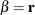whereis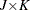andis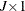, the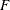-statistic with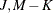degrees of freedom is computed as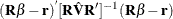However, it is also possible to write thestatistic as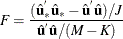where

•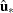is the residual vector from the restricted regression

•
is the residual vector from the unrestricted regression

•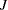is the number of restrictions

•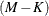are the degrees of freedom,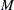is the number of observations, and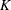is the number of parameters in the model

The Wald, likelihood ratio (LR) and Lagrange multiplier (LM) tests are all related to thetest. You use this relationship of thetest to the likelihood ratio and Lagrange multiplier tests. The Wald test is calculated from its definition.

The Wald test statistic is: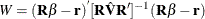The advantage of calculating Wald in this manner is that it enables you to substitute a heteroscedasticity-corrected covariance matrix for the matrix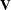. PROC PANEL makes such a substitution if you request the HCCME option in the MODEL statement.

The likelihood ratio is: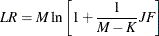The Lagrange multiplier test statistic is: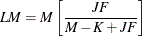where JF represents the number of restrictions multiplied by the result of thetest.

Note that only the Wald is changed when the HCCME option is selected. The LR and LM tests are unchanged.

The distribution of these test statistics is thewith degrees of freedom equal to the number of restrictions imposed (). The three tests are asymptotically equivalent, but they have differing small sample properties. Greene (2000, p. 392) and Davidson and MacKinnon (1993, pg. 456-458) discuss the small sample properties of these statistics.